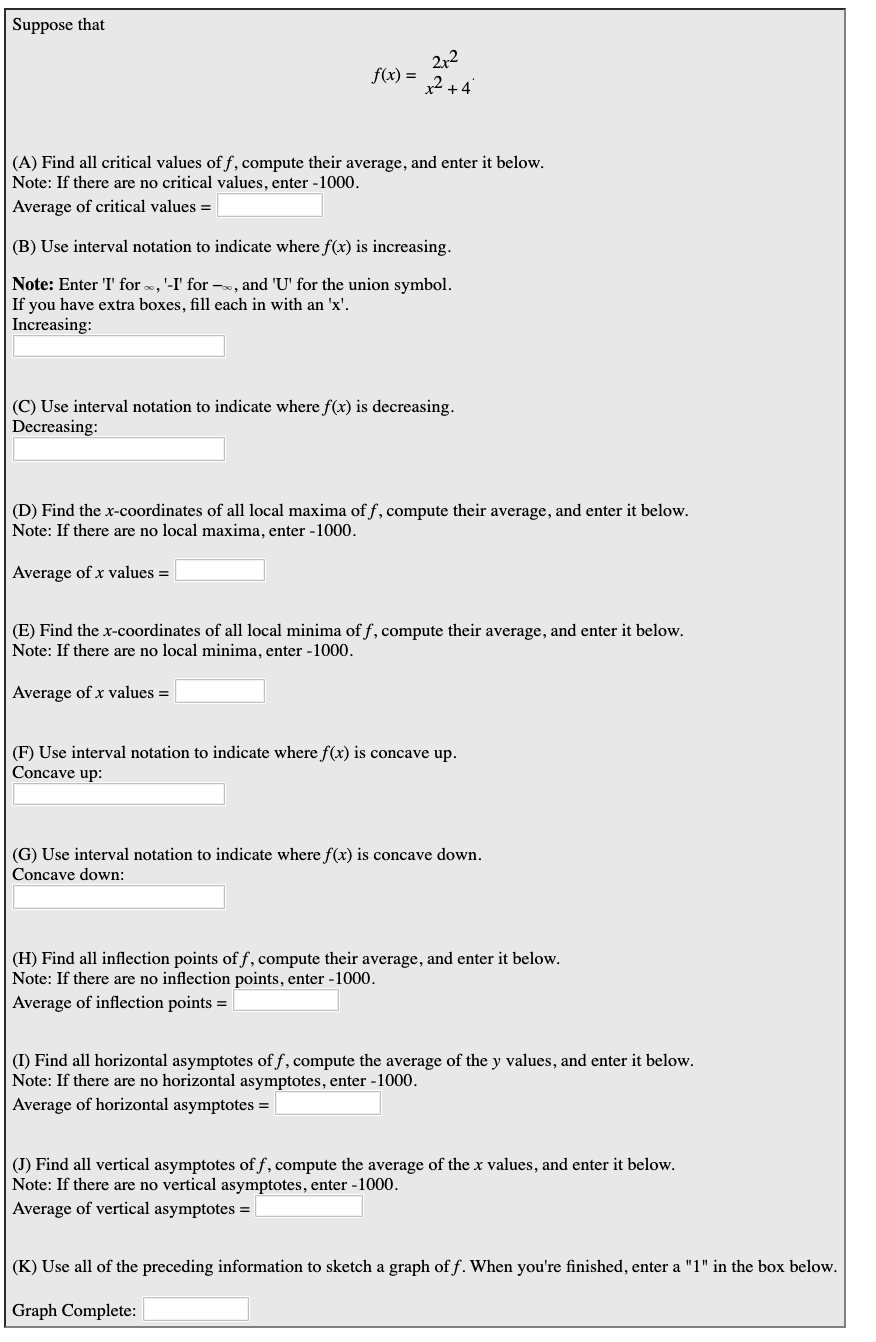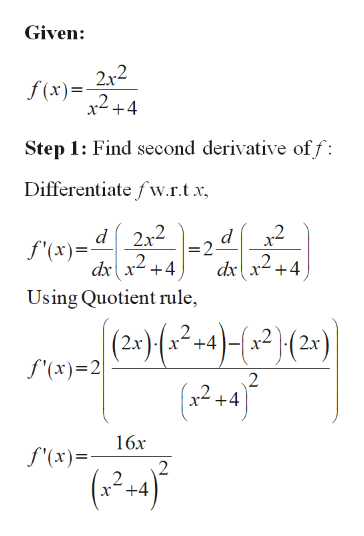# Suppose that212f(x)(A) Find all critical values of f, compute their average, and enter it below.Note: If there are no critical values, enter -1000Average of critical values =(B) Use interval notation to indicate where f(x) is increasing.Note: Enter T' for, '-I' for -, and 'U' for the union symbol.If you have extra boxes, fill each in with an 'x'Increasing:(C) Use interval notation to indicate where f(x) is decreasingDecreasing:(D) Find the x-coordinates of all local maxima of f, compute their average, and enter it below.Note: If there are no local maxima, enter -1000Average of x values =(E) Find the x-coordinates of all local minima of f, compute their average, and enter it belowNote: If there are no local minima, enter -1000.Average of x values =(F) Use interval notation to indicate where f(x) is concave up.Concave up:(G) Use interval notation to indicate where f(x) is concave down.Concave down(H) Find all inflection points off, compute their average, and enter it belowNote: If there are no inflection points, enter -1000Average of inflection points =(I) Find all horizontal asymptotes of f, compute the average of the y values, and enter it belowNote: If there are no horizontal asymptotes, enter -1000Average of horizontal asymptotes = |(J) Find all vertical asymptotes off, compute the average of the x values, and enter it belowNote: If there are no vertical asymptotes, enter -1000Average of vertical asymptotes = |(K) Use all of the preceding information to sketch a graph off. When you're finished, enter a "1" in the box below.Graph Complete:

Question
19 views

Section G,H,Ihelp_outlineImage TranscriptioncloseSuppose that 212 f(x) (A) Find all critical values of f, compute their average, and enter it below. Note: If there are no critical values, enter -1000 Average of critical values = (B) Use interval notation to indicate where f(x) is increasing. Note: Enter T' for, '-I' for -, and 'U' for the union symbol. If you have extra boxes, fill each in with an 'x' Increasing: (C) Use interval notation to indicate where f(x) is decreasing Decreasing: (D) Find the x-coordinates of all local maxima of f, compute their average, and enter it below. Note: If there are no local maxima, enter -1000 Average of x values = (E) Find the x-coordinates of all local minima of f, compute their average, and enter it below Note: If there are no local minima, enter -1000. Average of x values = (F) Use interval notation to indicate where f(x) is concave up. Concave up: (G) Use interval notation to indicate where f(x) is concave down. Concave down (H) Find all inflection points off, compute their average, and enter it below Note: If there are no inflection points, enter -1000 Average of inflection points = (I) Find all horizontal asymptotes of f, compute the average of the y values, and enter it below Note: If there are no horizontal asymptotes, enter -1000 Average of horizontal asymptotes = | (J) Find all vertical asymptotes off, compute the average of the x values, and enter it below Note: If there are no vertical asymptotes, enter -1000 Average of vertical asymptotes = | (K) Use all of the preceding information to sketch a graph off. When you're finished, enter a "1" in the box below. Graph Complete: fullscreen
check_circle

Step 1

Part (G):

Concave downward interval:

If F’’(x) <0, the function is concave down.help_outlineImage TranscriptioncloseGiven 2r2 f (x) x2+4 Step 1: Find second derivative off Differentiate fw.r.tx f(xd 2x2 d =2- +4 +4 Using Quotient rule (2t) (a2-n4)-2}(2) f(x)2 2 16x f'(x) (2-4 x°+4 fullscreen
Step 2

Now, differentiate f w.r.t x,

Step 3

Substitute f"...

### Want to see the full answer?

See Solution

#### Want to see this answer and more?

Solutions are written by subject experts who are available 24/7. Questions are typically answered within 1 hour.*

See Solution
*Response times may vary by subject and question.
Tagged in

### Functions